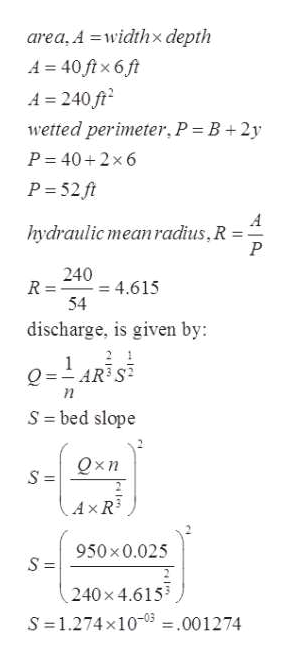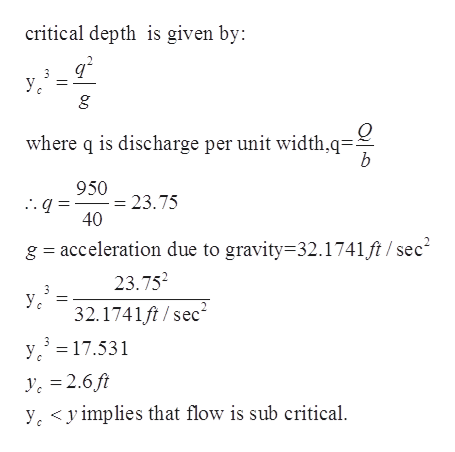# 1.) A 40-ft wide rectangular channel carries 950 ft3/sec at a depth of 6 ft. If n = 0.025, what must the channel slope be in order to produce uniform flow at the 6-ft depth? Is this flow subcritical or supercritical? What is the critical slope that would cause the flow to just flip to the other flow profile (subcritical → supercritical or supercritical → subcritical)?2.) Continuing with Problem 1:a. What is the alternate flow depth? (hint: in Problem 1 you should have found that flow was subcritical, so now you're finding the supercritical flow depth)b. What is the critical rise of the surface bed (Δzcrit) that would just cause the flow to flip to supercritical?c. What is the critical width (bcrit) of a throat in a contraction of the channel that would just cause the flow to switch to supercritical?

Question
37 views

1.) A 40-ft wide rectangular channel carries 950 ft3/sec at a depth of 6 ft. If n = 0.025, what must the channel slope be in order to produce uniform flow at the 6-ft depth? Is this flow subcritical or supercritical? What is the critical slope that would cause the flow to just flip to the other flow profile (subcritical → supercritical or supercritical → subcritical)?

2.) Continuing with Problem 1:
a. What is the alternate flow depth? (hint: in Problem 1 you should have found that flow was subcritical, so now you're finding the supercritical flow depth)
b. What is the critical rise of the surface bed (Δzcrit) that would just cause the flow to flip to supercritical?
c. What is the critical width (bcrit) of a throat in a contraction of the channel that would just cause the flow to switch to supercritical?

check_circle

Step 1

note: since you you have not mentioned which question to solve ,we are providing the solution of question 1 as per our guidlines. please upload other question for getting the solution.

Given:

Width of the rectangular channel=40ft

Discharge, Q= 950 ft3/sec

n= 0.025

Depth = 6ft

To find: channel slope

Step 2

to find the slope of bed :help_outlineImage Transcriptionclosearea, A widthx depth A 40 ftx 6 ft A 240 t wetted perimeter, P = B + 2y P 40+2x6 P 52 ft hydraulic meanradius, R = P 240 - 4.615 54 R = discharge, is given by: 2 1 Q =AR S S = bed slope Qxn S = AXR3 950 x0.025 240x 4.615 S 1.274x10 001274 fullscreen
Step 3

to determine if the flow is sub c...help_outlineImage Transcriptionclosecritical depth is given by: У, g where q is discharge per unit width.q= b 950 = 23.75 40 g acceleration due to gravity 32.1741ft / sec 23.752 У. 32.1741ft/sec2 y3 17.531 ye 2.6ft yyimplies that flow is sub critical fullscreen

### Want to see the full answer?

See Solution

#### Want to see this answer and more?

Solutions are written by subject experts who are available 24/7. Questions are typically answered within 1 hour.*

See Solution
*Response times may vary by subject and question.
Tagged in

### Civil Engineering